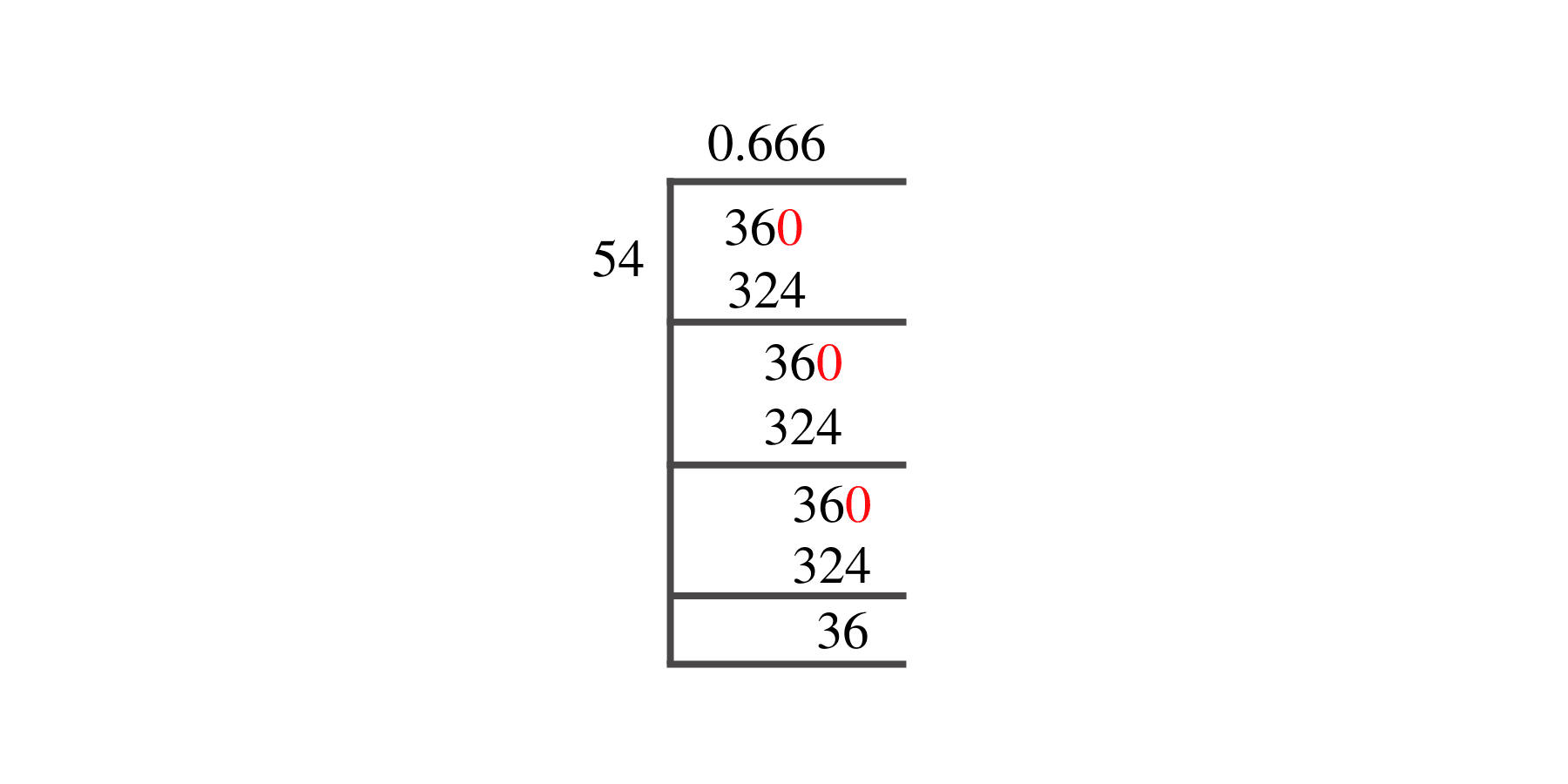# What Is 36/54 as a Decimal + Solution With Free Steps

The fraction 36/54 as a decimal is equal to 0.666.

If a digit or group of digits in the decimal component of a decimal number infinitely repeats itself then, these decimals are referred to as recurring decimals or non-terminating decimals. The given fraction of 36/54 is equivalent to 0.666, which is a recurring decimal.

Here, we are more interested in the division types that result in a Decimal value, as this can be expressed as a Fraction. We see fractions as a way of showing two numbers having the operation of Division between them that result in a value that lies between two Integers.Now, we introduce the method used to solve said fraction to decimal conversion, called Long Division, which we will discuss in detail moving forward. So, let’s go through the Solution of fraction 36/54.

## Solution

First, we convert the fraction components, i.e., the numerator and the denominator, and transform them into the division constituents, i.e., the Dividend and the Divisor, respectively.

This can be done as follows:

Dividend = 36

Divisor = 54

Now, we introduce the most important quantity in our division process: the Quotient. The value represents the Solution to our division and can be expressed as having the following relationship with the Division constituents:

Quotient = Dividend $\div$ Divisor = 36 $\div$ 54

This is when we go through the Long Division solution to our problem, as shown in figure 1.

##36/54 Long Division Method

We start solving a problem using the Long Division Method by first taking apart the division’s components and comparing them. As we have 36 and 54, we can see how 36 is Smaller than 54, and to solve this division, we require that 36 be Bigger than 54.

This is done by multiplying the dividend by 10 and checking whether it is bigger than the divisor or not. If so, we calculate the Multiple of the divisor closest to the dividend and subtract it from the Dividend. This produces the Remainder, which we then use as the dividend later.

Now, we begin solving for our dividend 36, which after getting multiplied by 10 becomes 360.

We take this 360 and divide it by 54; this can be done as follows:

360 $\div$ 54 $\approx$ 6

Where:

54 x 6 = 324

This will lead to the generation of a Remainder equal to 360 – 320 = 36. Now this means we have to repeat the process by Converting the 36 into 360 and solving for that:

360 $\div$ 54 $\approx$ 6

Where:

54 x 6 = 324

This, therefore, again produces  Remainder which is equal to 360 – 324 = 36. Now we must solve this problem to Third Decimal Place for accuracy, so we repeat the process with the same dividend 360.

360 $\div$ 54 $\approx$ 6

Where:

54 x 6 = 324

Therefore, we have a Quotient generated after combining the three pieces of it as 0.666=z, with a Remainder equal to 36.Images/mathematical drawings are created with GeoGebra.